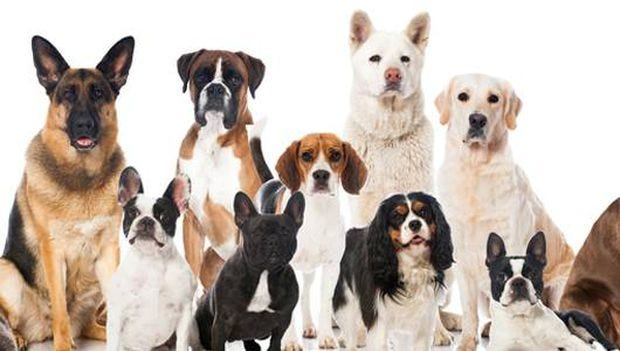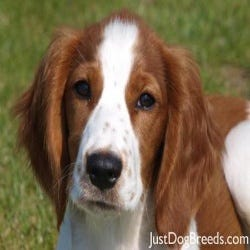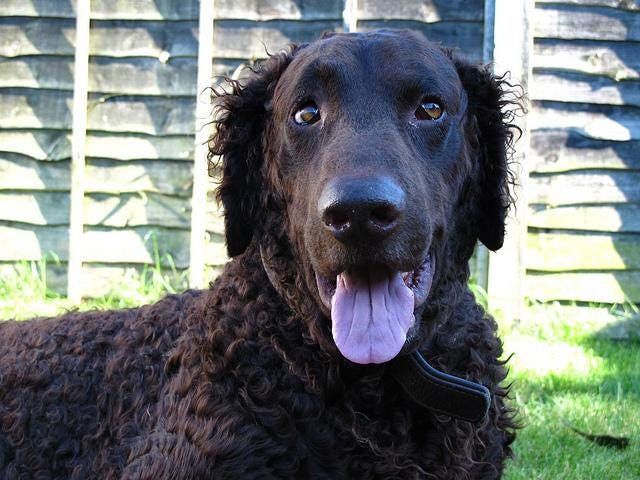# Dog Breed Classification using PyTorch

Source: Deep Learning on Medium

Github repository for Dog Breed Classification.

Today we are going to take our first step to build a Shazam like application. Though, our goal is not to detect songs but to detect dog breeds. This dataset provides the images of 133 different dog breeds. At the end of this project, our code will accept any user-supplied image as input. If a dog is detected in the image, it will provide an estimate of the dog’s breed. If a human is detected, it will provide an estimate of the dog breed that is most resembling.

It is important to mention that the task of assigning breed to dogs from images is considered exceptionally challenging. To see why, consider that even a human would have trouble distinguishing between a Brittany and a Welsh Springer Spaniel.Brittany — — — — — — — — — — — — — — — — — — — — — — — — — — — — Welsh Springer Spaniel

Likewise, recall that Labradors come in yellow, chocolate, and black.It is not difficult to find other dog breed pairs with minimal inter-class variation (for instance, Curly-Coated Retrievers and American Water Spaniels).American water spaniel — — — — — — — — — — — — — — — — — — — — — — — — — — — — — — Curly-Coated Retrievers

We will first write a CNN model in PyTorch to see how our model classifies the dog breeds. We need to write image transformations and loaders. We are going to resize the images to 224×224. As the color information is important we are going to use all color channels for the image. Input tensor shape will be 224x224x3. We will augment the training set by flipping the images horizontally and rotating the images for 10 degree.

`data_dir = '/data/dog_images'train_dir = data_dir + '/train'valid_dir = data_dir + '/valid'test_dir = data_dir + '/test'`
`# Image Transformationdata_transforms = {    'train' : transforms.Compose([    transforms.Resize(224),    transforms.RandomHorizontalFlip(), # randomly flip and rotate    transforms.RandomRotation(10),    transforms.ToTensor(),    transforms.Normalize((0.5, 0.5, 0.5), (0.5, 0.5, 0.5))    ]),        'valid' : transforms.Compose([    transforms.Resize(224),    transforms.ToTensor(),    transforms.Normalize((0.5, 0.5, 0.5), (0.5, 0.5, 0.5))    ]),        'test' : transforms.Compose([    transforms.Resize(224),    transforms.ToTensor(),    transforms.Normalize((0.5, 0.5, 0.5), (0.5, 0.5, 0.5))    ]),}`
`# Reading Datasetimage_datasets = {    'train' : ImageFolder(root=train_dir,transform=data_transforms['train']),    'valid' : ImageFolder(root=valid_dir,transform=data_transforms['valid']),    'test' : ImageFolder(root=test_dir,transform=data_transforms['test'])}`
`# Loading Datasetdata_loaders = {    'train' : DataLoader(image_datasets['train'],batch_size = batch_size,shuffle=True),    'valid' : DataLoader(image_datasets['valid'],batch_size = batch_size),    'test' : DataLoader(image_datasets['test'],batch_size = batch_size)    }`

After running the code above, we can also find out the size of training, validation, testing datasets and number of classes(dog breeds).

`Size of training set is: 6680Size of validation set is: 835Size of testing set is: 836`
`Number of classes are: 133`

To define the model architecture:

• We used the six convolution layers all with the colvolution of size = 3, stride = 1 and padding = 0
• Relu activations are used after each convoltution layers except the last one.
• Max pooling layers of 2×2 are applied.
• Batch normalization is applied after each max pool layer.
• Dropout is applied with the probability of 0.2.

These choices are made after following an iterative process of improving validation accuracy

`# defining the CNN architectureclass Net(nn.Module):        def __init__(self):        super(Net, self).__init__()        self.conv1 = nn.Conv2d(3, 16, 3)        self.conv2 = nn.Conv2d(16, 32, 3)        self.conv3 = nn.Conv2d(32, 64, 3)        self.conv4 = nn.Conv2d(64, 128, 3)        self.conv5 = nn.Conv2d(128, 256, 3)`
`self.fc1 = nn.Linear(256 * 6 * 6, 133)                self.max_pool = nn.MaxPool2d(2, 2,ceil_mode=True)        self.dropout = nn.Dropout(0.2)`
`self.conv_bn1 = nn.BatchNorm2d(224,3)        self.conv_bn2 = nn.BatchNorm2d(16)        self.conv_bn3 = nn.BatchNorm2d(32)        self.conv_bn4 = nn.BatchNorm2d(64)        self.conv_bn5 = nn.BatchNorm2d(128)        self.conv_bn6 = nn.BatchNorm2d(256)        def forward(self, x):                x = F.relu(self.conv1(x))        x = self.max_pool(x)        x = self.conv_bn2(x)                x = F.relu(self.conv2(x))        x = self.max_pool(x)        x = self.conv_bn3(x)                x = F.relu(self.conv3(x))        x = self.max_pool(x)        x = self.conv_bn4(x)                x = F.relu(self.conv4(x))        x = self.max_pool(x)        x = self.conv_bn5(x)                x = F.relu(self.conv5(x))        x = self.max_pool(x)        x = self.conv_bn6(x)                x = x.view(-1, 256 * 6 * 6)                x = self.dropout(x)        x = self.fc1(x)`
`return x`
`# instantiate the CNNmodel_scratch = Net()`
`# move tensors to GPU if CUDA is availableif use_cuda:    model_scratch.cuda()`

We also defined functions to train and test the model:

`def train(n_epochs, loaders, model, optimizer,scheduler, criterion, use_cuda, save_path):    """returns trained model"""    # initialize tracker for minimum validation loss    valid_loss_min = np.Inf         for epoch in range(1, n_epochs+1):        # initialize variables to monitor training and validation loss        train_loss = 0.0        valid_loss = 0.0                ###################        # train the model #        ###################        scheduler.step()        model.train()        for batch_idx, (data, target) in enumerate(loaders['train']):            # move to GPU            if use_cuda:                data, target = data.cuda(), target.cuda()            ## find the loss and update the model parameters accordingly            # clear the gradients of all optimized variables            optimizer.zero_grad()            # forward pass: compute predicted outputs by passing inputs to the model            output = model(data)            # calculate the batch loss            loss = criterion(output, target)            # backward pass: compute gradient of the loss with respect to model parameters            loss.backward()            # perform a single optimization step (parameter update)            optimizer.step()            # update training loss            ## record the average training loss, using something like            train_loss = train_loss + ((1 / (batch_idx + 1)) * (loss.data - train_loss))                    ######################            # validate the model #        ######################        model.eval()        for batch_idx, (data, target) in enumerate(loaders['valid']):            # move to GPU            if use_cuda:                data, target = data.cuda(), target.cuda()            ## update the average validation loss             # forward pass: compute predicted outputs by passing inputs to the model            output = model(data)            # calculate the batch loss            loss = criterion(output, target)            # update average validation loss             valid_loss = valid_loss + ((1 / (batch_idx + 1)) * (loss.data - valid_loss))`
`# calculate average losses        train_loss = train_loss/len(train_loader.dataset)        valid_loss = valid_loss/len(valid_loader.dataset)`
`# print training/validation statistics         print('Epoch: {} \tTraining Loss: {:.6f} \tValidation Loss: {:.6f}'.format(            epoch,             train_loss,            valid_loss            ))                ## save the model if validation loss has decreased        if valid_loss <= valid_loss_min:            print('Validation loss decreased ({:.6f} --> {:.6f}).  Saving model ...'.format(            valid_loss_min,            valid_loss))            torch.save(model.state_dict(), save_path)            valid_loss_min = valid_loss        # return trained model    return model`

Test Function:

`def test(loaders, model, criterion, use_cuda):`
`# monitor test loss and accuracy    test_loss = 0.    correct = 0.    total = 0.`
`model.eval()    for batch_idx, (data, target) in enumerate(loaders['test']):        # move to GPU        if use_cuda:            data, target = data.cuda(), target.cuda()        # forward pass: compute predicted outputs by passing inputs to the model        output = model(data)        # calculate the loss        loss = criterion(output, target)        # update average test loss         test_loss = test_loss + ((1 / (batch_idx + 1)) * (loss.data - test_loss))        # convert output probabilities to predicted class        pred = output.data.max(1, keepdim=True)        # compare predictions to true label        correct += np.sum(np.squeeze(pred.eq(target.data.view_as(pred))).cpu().numpy())        total += data.size(0)                print('Test Loss: {:.6f}\n'.format(test_loss))`
`print('\nTest Accuracy: %2d%% (%2d/%2d)' % (        100. * correct / total, correct, total))`

We use the Adam Optimizer with a learning rate of 0.001. We trained the model for 20 epochs and achieved the test accuracy of 26%.

As the accuracy is very low, we tried the transfer learning approach. We used the resnet model for the transfer learning approach.

`model_transfer = models.resnet18(pretrained=True)for param in model_transfer.parameters():    param.requires_grad = False`
`num_ftrs = model_transfer.fc.in_featuresmodel_transfer.fc = nn.Linear(num_ftrs, 133)`
`# if GPU is available, move the model to GPUif use_cuda:    model_transfer.cuda()`

After training the model for 20 epochs, we achieved the test accuracy of 71% which is a significant improvement from our first try. Here, we just started to find a dog breed classification solution, next we will make improvements in our approach to achieve better accuracy. Some of the possible experiments to conduct are:

• Trying to clean the dataset. The images are very noisy as in some of the images have human faces beside the dogs, hands or other noise features like different background colors. Another problem is that images have different dogs in different poses which makes it difficult for the classifier to learn.